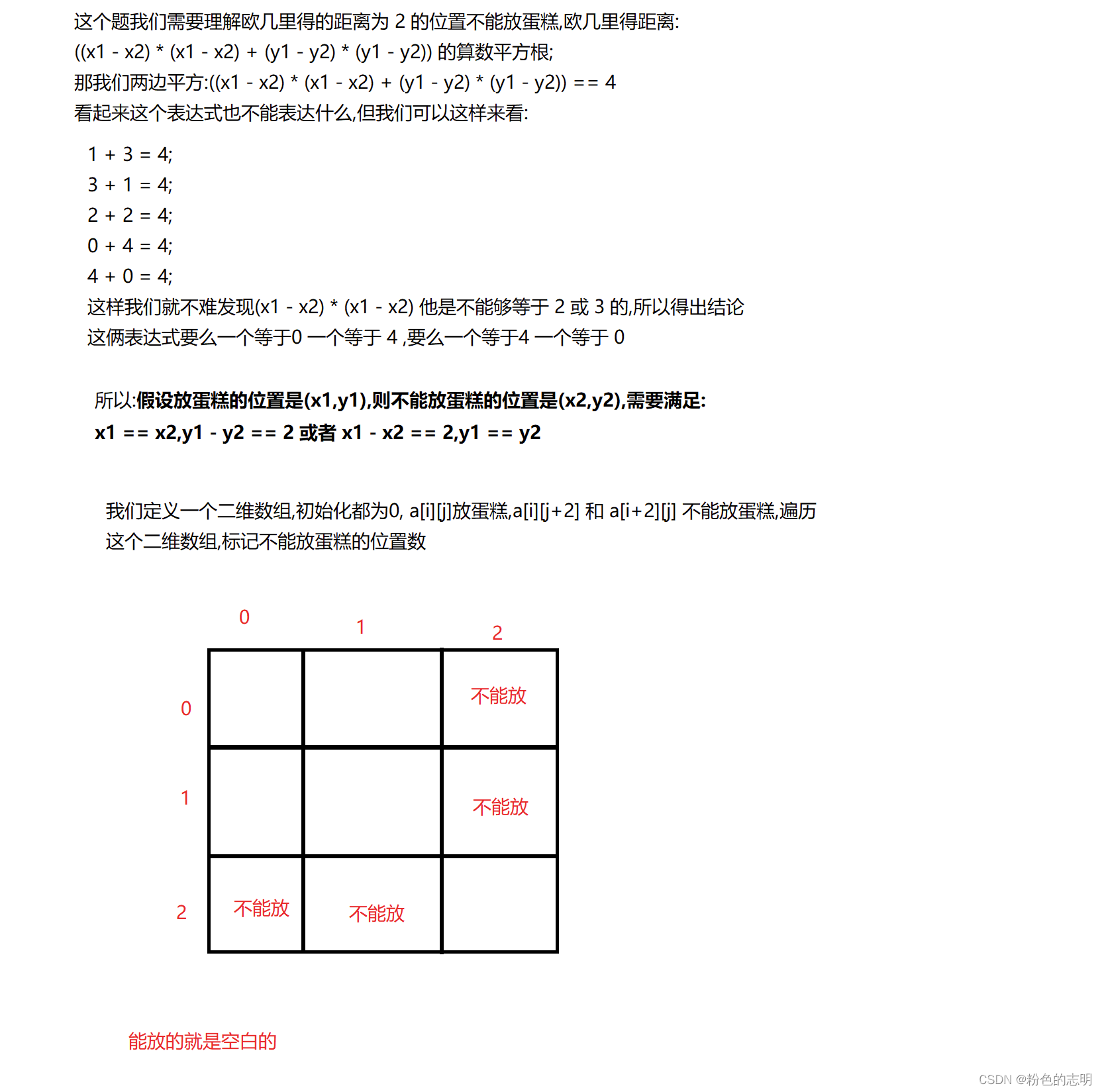# 不要二

( (x1-x2) * (x1-x2) + (y1-y2) * (y1-y2) ) 的算术平方根

## 解题思路## 解题代码

``````import java.util.*;
public class Main{

public static void main(String[] args){

Scanner scan = new Scanner(System.in);
int w = scan.nextInt();
int h = scan.nextInt();
int[][] array = new int[w][h];
int count = 0;
for(int i = 0;i < w;i++){

for(int j = 0;j < h;j++){

if(array[i][j] == 0){

count++;
if(i + 2 < w){

//不能放的置为 1
array[i + 2][j] = 1;
}
if(j + 2 < h){

//不能放的置为 1
array[i][j + 2] = 1;
}
}

}
}
System.out.println(count);
}
}
``````

# 字符串转整数

`注意：`
①字符串中可能出现任意符号，出现除 +/- 以外符号时直接输出 0
②字符串中可能出现 +/- 且仅可能出现在字符串首位。

“+2147483647”

2147483647

“1a33”

0

## 解题思路

1:空字符串
2:正负号处理
3:数字串中存在非法字符

int sum = 0;
sum * 10 + 1 = 1;
sum * 10 + 2 = 12;
sum * 10 + 3 = 123;

## 解题代码

``````public class Solution {

public int StrToInt(String str) {

//先转换成字符数组
char[] arr = str.toCharArray();
if(str.isEmpty()){

return 0;
}
//判断是否为负
int ret = 1;
if(arr == '-'){

ret = -1;
arr = '0';
}else if(arr == '+'){

ret = 1;
arr = '0';
}

//记录该数
int sum = 0;
for(int i = 0;i < arr.length;i++){

//利用ascli码值判断是否为数字,不为数字,直接让sum = 0,退出循环
if(arr[i] < '0' || arr[i] > '9'){

sum = 0;
break;
}
//说明为数字,每次从高位加着走,让字符减 '0' 获得数字的大小
sum = sum * 10 + arr[i] - '0';
}
//如果ret = 1,返回正数,如果为 -1,返回负数
return ret * sum;
}
}
``````